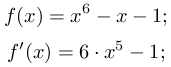# Entries tagged code

## PHP + Laravel development Packages for atom.io

Posted on 30. Januar 2018 Comments

## PHP CS Fixer

`brew install php-cs-fixer`

`apm install php-cs-fixer`

Settings:

php path: `/usr/local/bin/php`

php-cs-fixer path: `/usr/local/bin/php-cs-fixer`

PHP CS Fixer Rules: `@PSR2,blank_line_after_opening_tag,whitespace_after_comma_in_array,blank_line_after_namespace`

PHP CS Fixer Arguments: `--using-cache=no, --no-interaction, --stop-on-violation`

Ctrl+Cmd+S

Can also be used on commandline for folders, e.g.
`\$ php-cs-fixer fix app/ --rules=@PSR2,blank_line_after_opening_tag,whitespace_after_comma_in_array,blank_line_after_namespace`

## php-integrator-refactoring (php-integrator-base)

`apm install php-integrator-base`

`apm install php-integrator-refactoring`

Linting (also see linter(-php))

Sort use statements

## linter and linter-php

`apm install linter`

`apm install linter-php`

In case linting with php-integrator-base does not work.

`apm install language-blade`

`apm install language-vue`

## minimap

`apm install minimap`

Short overview over the code on the right side

## highlight-selected

`apm install highlight-selected`

## platform-ide-terminal

`apm install platform-ide-terminal`

Instead of switching windows, terminal right in atom

Cmd+Shift+T

## symbols-tree-view

`apm install symbols-tree-view`

Structured overview on the right side over classes, constants, attributes, methods etc

## hyperclick and hyperclick-php

`apm install hyperclick`

`apm install hyperclick-php`

Cmd+Click on classes, functions etc to go to origin.

Not needed if you installed `atom-ide-ui`

## teletype

`apm install teletype`

Work on the same file with coworkers, encrypted via WebRTC

More dependencies might be installed with installing some of these packages

## Berechnen von Nullstellen in Matlab

Posted on 30. Juli 2011 Comments

Als Beispiel möchte ich hier die Funktion und ihre Ableitung benutzen:• a, b: Intervall
• eta: Genauigkeit
• format long: 15 Nachkommastellen

//update: GitHub

//update: Nullstellenberechnung in Lua

1. Bisektion (Wikipedia)

```function [nullstelle,i]=bisektion(a,b)
format long;
eta = 0.00001;
i=0;
if(f(b)*f(a)<0)
while(abs(b-a)>eta)
if(f((a+b)/2)*f(a)>0)
a=(a+b)/2;
else
b=(a+b)/2;
end
i=i+1;
end
nullstelle=(a+b)/2;
end

function f_x=f(x)
f_x=x.^6-x-1;```

2. Newtown-Verfahren

```function [nullstelle,i]=newton_verfahren(x)
format long;
eta=0.00001;
diff=1;
i=0;
while(diff>eta)
nullstelle = x;
[f_x,fp_x]=f(x);
x=x-f_x/fp_x;
i=i+1;
diff=abs(x-nullstelle);
end
nullstelle=x;

function [f_x,fp_x]=f(x)
f_x  = x.^6-x-1;
fp_x = 6*x.^5-1;```

3. Sekanten-Verfahren

```function [nullstelle,i]=sekanten_verfahren(a,b)
format long;
eta=0.00001;
i=0;
while(abs(b-a)>eta)
nullstelle=b;
b=b-f(b)*(b-a)/(f(b)-f(a));
a=nullstelle;
i=i+1;
end
nullstelle=(a+b)/2;

function f_x=f(x)
f_x=x.^6-x-1;```

4. Regular-Falsi

```function [nullstelle,i]=regula_falsi(a,b)
format long;
eta=0.00001;
i=0;
diff=1;
if(f(b)*f(a)<0)
nullstelle = a;
while(diff>eta)
x=(a*f(b)-b*f(a))/(f(b)-f(a));
if(f(x)<0)
a=x;
else
b=x;
end
diff=abs(x-nullstelle);
nullstelle = x;
i=i+1;
end
end

function f_x=f(x)
f_x=x.^6-x-1;```